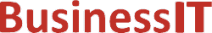O nás     Inzerce     KontaktSpolehlivé informace o IT již od roku 2011[Linux manuál]

# fma, fmaf, fmal: plovoucí desetinná čárka násobit-přidat

Originální popis anglicky: fma, fmaf, fmal - floating-point multiply-add

Návod, kniha: POSIX Programmer's Manual

## STRUČNĚ

#include <math.h>

double fma(double x, double y, double z);

float fmaf(float x, float y, float z);

long double fmal(long double x, long double y, long double z);

## POPIS / INSTRUKCE

These functions shall compute ( x * y) +  z, rounded as one ternary operation: they shall compute the value (as if) to infinite precision and round once to the result format, according to the rounding mode characterized by the value of FLT_ROUNDS.
An application wishing to check for error situations should set errno to zero and call feclearexcept(FE_ALL_EXCEPT) before calling these functions. On return, if errno is non-zero or fetestexcept(FE_INVALID | FE_DIVBYZERO | FE_OVERFLOW | FE_UNDERFLOW) is non-zero, an error has occurred.

## NÁVRATOVÁ HODNOTA

Upon successful completion, these functions shall return ( x *  y) + z, rounded as one ternary operation.
If x or y are NaN, a NaN shall be returned.
If x multiplied by y is an exact infinity and z is also an infinity but with the opposite sign, a domain error shall occur, and either a NaN (if supported), or an implementation-defined value shall be returned.
If one of x and y is infinite, the other is zero, and z is not a NaN, a domain error shall occur, and either a NaN (if supported), or an implementation-defined value shall be returned.
If one of x and y is infinite, the other is zero, and z is a NaN, a NaN shall be returned and a domain error may occur.
If x* y is not 0*Inf nor Inf*0 and z is a NaN, a NaN shall be returned.

## CHYBY / ERRORY

These functions shall fail if:
Domain Error
The value of x* y+ z is invalid, or the value x* y is invalid and z is not a NaN.
If the integer expression (math_errhandling & MATH_ERRNO) is non-zero, then errno shall be set to [EDOM]. If the integer expression (math_errhandling & MATH_ERREXCEPT) is non-zero, then the invalid floating-point exception shall be raised.
Range Error
The result overflows.
If the integer expression (math_errhandling & MATH_ERRNO) is non-zero, then errno shall be set to [ERANGE]. If the integer expression (math_errhandling & MATH_ERREXCEPT) is non-zero, then the overflow floating-point exception shall be raised.

These functions may fail if:
Domain Error
The value x* y is invalid and z is a NaN.
If the integer expression (math_errhandling & MATH_ERRNO) is non-zero, then errno shall be set to [EDOM]. If the integer expression (math_errhandling & MATH_ERREXCEPT) is non-zero, then the invalid floating-point exception shall be raised.
Range Error
The result underflows.
If the integer expression (math_errhandling & MATH_ERRNO) is non-zero, then errno shall be set to [ERANGE]. If the integer expression (math_errhandling & MATH_ERREXCEPT) is non-zero, then the underflow floating-point exception shall be raised.

The following sections are informative.

None.

## APPLICATION USAGE

On error, the expressions (math_errhandling & MATH_ERRNO) and (math_errhandling & MATH_ERREXCEPT) are independent of each other, but at least one of them must be non-zero.

## RATIONALE

In many cases, clever use of floating ( fused) multiply-add leads to much improved code; but its unexpected use by the compiler can undermine carefully written code. The FP_CONTRACT macro can be used to disallow use of floating multiply-add; and the fma() function guarantees its use where desired. Many current machines provide hardware floating multiply-add instructions; software implementation can be used for others.

None.

## SOUVISEJÍCÍ

feclearexcept() , fetestexcept() , the Base Definitions volume of IEEE Std 1003.1-2001, Section 4.18, Treatment of Error Conditions for Mathematical Functions, <math.h> Portions of this text are reprinted and reproduced in electronic form from IEEE Std 1003.1, 2003 Edition, Standard for Information Technology -- Portable Operating System Interface (POSIX), The Open Group Base Specifications Issue 6, Copyright (C) 2001-2003 by the Institute of Electrical and Electronics Engineers, Inc and The Open Group. In the event of any discrepancy between this version and the original IEEE and The Open Group Standard, the original IEEE and The Open Group Standard is the referee document. The original Standard can be obtained online at http://www.opengroup.org/unix/online.html .
 2003 IEEE/The Open Group
©2011-2020 BusinessIT.cz, ISSN 1805-0522 | Názvy použité v textech mohou být ochrannými známkami příslušných vlastníků.
Provozovatel: Bispiral, s.r.o., kontakt: BusinessIT(at)Bispiral.com | Inzerce: Best Online Media, s.r.o., zuzana@online-media.cz
O vydavateli | Pravidla webu BusinessIT.cz a ochrana soukromí | pg(8905)